﻿

### A class of two-dimensional translation planes admitting SL(2,5)

#### Abstract

A class of translation planes of order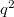$q^2$ admitting SL(2,5) is described, constructed from replaceable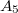$A_5$-invariant nests of reguli in a regular spread of PG(3,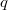$q$), where$q$ is odd and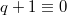$q+1\equiv0$ (mod 5). The translation planes of order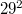$29^2$ in this class are determined.

DOI Code: 10.1285/i15900932v29n1supplp223

Keywords:
Translation planes; nest replacement; SL(2,5)

Full Text: PDF